# Capacitors in Series and Parallel

## Capacitor in Series

Let us connect n number of capacitors in series. V volt is applied across this series combination of capacitors.Let us consider capacitance of capacitors are C1, C2, C3…….Cn respectively, and equivalent capacitance of series combination of the capacitors is C. The voltage drops across capacitors are considered to be V1, V2, V3…….Vn, respectively.Now, if Q coulomb be the charge transferred from the source through these capacitors, then,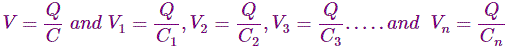Since the charge accumulated in each capacitor and I entire series combination of capacitors will be same and it is considered as Q.
Now, equation (i) can be written as,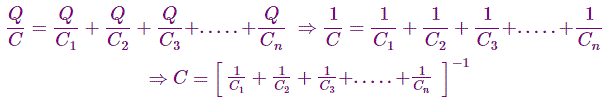## Capacitors in Parallel

A capacitor is designed to store the energy in the form of its electric field, i.e. electrostatic energy. Whenever there is a necessity to increase more electrostatic energy storing capacity, a suitable capacitor of increased capacitance is required. A capacitor is made up of two metal plates connected in parallel and separated by a dielectric medium like glass, mica, ceramics etc. The dielectric provides a non-conducting medium between the plates and has a unique ability to hold the charge, and the ability of the capacitor to store charge is defined as the capacitance of the capacitor. When a voltage source is connected across the plates of the capacitor a positive charge on one plate, and negative charge on the other plate get deposited. The total amount of charge (q) accumulated is directly proportional to the voltage source (V) such that,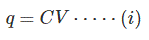Where, C is proportionality constant i.e. capacitance. Its value depends upon physical dimensions of the capacitor.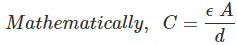Where ε = dielectric constant, A = effective plate area and d = space between plates.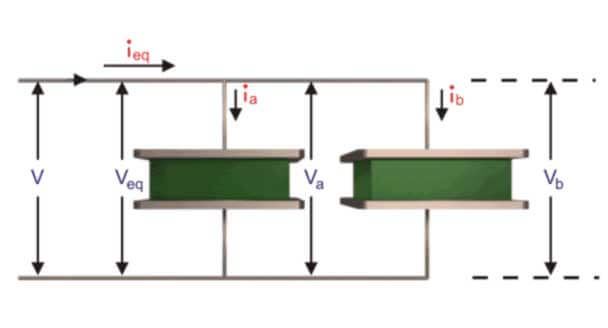To increase the capacitance value of a capacitor, two or more capacitors are connected in parallel as two similar plates joined together joined together, then their effective overlapping area is added with constant spacing between them and hence their equivalent capacitance value becomes double (C ∝ A) of individual capacitance. The capacitor bank is utilized in various manufacturing and processing industries incorporates capacitor in parallel, so to provide a capacitance of desired value as required by regulating the connection of capacitors connected in parallel and thus it is utilized efficiently as a static compensator for the reactive power balance in power system compensation. When two capacitors are connected in parallel then the voltage (V) across each capacitor is same i.e. (Veq = Va = Vb) and current( ieq ) is divided into two parts ia and ib. As it is known that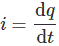Putting the value of q from equation (1) in the above equation,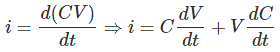The later term becomes zero (as capacitor’ capacitance is constant). Therefore,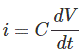Applying Kirchhoff’s Current Law at the incoming node of the parallel connection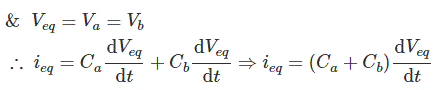Finally we get,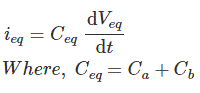Hence, whenever n capacitors are connected in parallel the equivalent capacitance of the whole connection is given by following equation which resembles similar to the equivalent resistance of resistors when connected in series.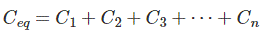#### Method of Finding Expression of Equivalent Capacitance of Parallel Capacitor

Let us connect n number of capacitors in parallel, across a voltage source of V volt.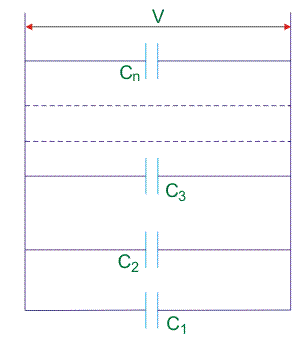Let us consider the capacitance of the capacitors are C1, C2, C3…..Cn, respectively and equivalent capacitance of the combination of the capacitor is C. As the capacitors are connected in parallel, like current charge in each capacitor will be same. Total charge of the parallel combination, will be divided in each capacitor according to it’s capacitance value but voltage across each capacitor will be same and at steady state condition it is exactly equal to the applied voltage.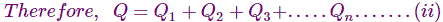Where,Q1, Q2, Q3,…….Qn are the charge of capacitor C1, C2, C3….. Cn respectively.Now equation (2) can be written as,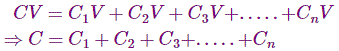Want To Learn Faster? 🎓
Get electrical articles delivered to your inbox every week.
No credit card required—it’s 100% free.